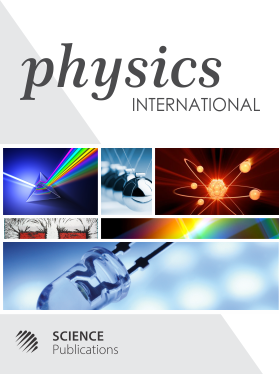Frequency: Continuous
ISSN: 1948-9803 (Print)
ISSN: 1948-9811 (Online)
Research Article Open Access

# Possible Physical Determination of the Mass, Size, Doubling Time and Density of the Unicellular Organisms Based on the Fundamental Physical Constants

Atanas Todorov Atanasov1
• 1 Trakia University, Bulgaria
Physics International
Volume 7 No. 2, 2016, 35-43

DOI:

Submitted On: 15 July 2016 Published On: 20 November 2016

How to Cite: Atanasov, A. T. (2016). Possible Physical Determination of the Mass, Size, Doubling Time and Density of the Unicellular Organisms Based on the Fundamental Physical Constants. Physics International, 7(2), 35-43. https://doi.org/10.3844/pisp.2016.35.43

## Abstract

In manuscript the hypothesis 'that the mass, size, doubling time and density of the unicellular organisms (Prokaryotes and Eukaryotes) are determined by the gravitational constant (G, N·m2/kg2), Planck constant (h, J·s) and growth rate vgr (m/s)' is investigated. By scaling analyses it is indicated that the growth rate of the unicellular organisms ranges in a narrow window of 1.0×10-11–1.0×10-10 m/s, in comparison to 10 orders of magnitudes difference between their mass. Dimension analyses demonstrates that the combination between the growth rate of unicellular organisms, gravitational constant and Planck constant provides the equations with dimension of mass M(vgr) = (h·vgr/G)½ in kilogram, length L(vgr) = (h·G/vgr3)½ in meter, time T(vgr) = (h·G/vgr5)½ in seconds and density ρ = vgr.3.5/hG2 in kg per 1 m3. For values of growth rate in numerical diapason of 1.0×10-11–1.0×10-9.5 m/s, the calculated numerical values for mass (3.0×10-18–1.0×10-16 kg), length (5.0×108–1.0×10-5 m), time (1.0×102–1.0×106 s) and density (1.0×10-1–1.0×104 kg/m3) overlap with diapason of experimentally measured values for cell mass (3.0×10-18–1.0×10-15 kg), volume to surface ratio (1.0×10-7–1.0×10-4 m), doubling time (1.0×103–1.0×107 s) and density (1050-1300 kg/m3) in both bacteria and protozoa.

• 2,101 Views